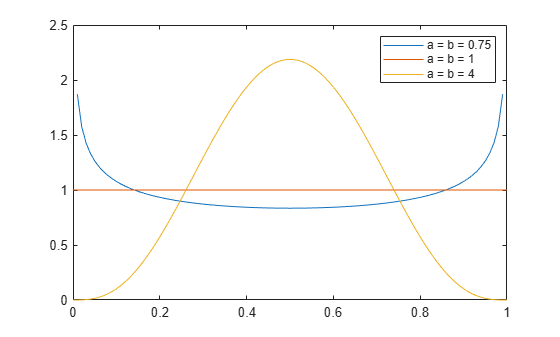## Beta Distribution

### Overview

The beta distribution describes a family of curves that are nonzero only on the interval [0,1]. A more general version of the function assigns parameters to the endpoints of the interval.

Statistics and Machine Learning Toolbox™ provides several ways to work with the beta distribution. You can use the following approaches to estimate parameters from sample data, compute the pdf, cdf, and icdf, generate random numbers, and more.

• Fit a probability distribution object to sample data, or create a probability distribution object with specified parameter values. See `Using` `BetaDistribution` `Objects` for more information.

• Work with data input from matrices, tables, and dataset arrays using probability distribution functions. See Supported Distributions for a list of beta distribution functions.

• Interactively fit, explore, and generate random numbers from the distribution using an app or user interface.

For more information on each of these options, see Working with Probability Distributions.

### Parameters

The beta distribution uses the following parameters.

ParameterDescriptionSupport
`a`First shape parameter$a>0$
`b`Second shape parameter$b>0$

### Probability Density Function

The probability density function (pdf) of the beta distribution is

`$y=f\left(x|a,b\right)=\frac{1}{B\left(a,b\right)}{x}^{a-1}{\left(1-x\right)}^{b-1}{I}_{\left[0,1\right]}\left(x\right)$`

where B( · ) is the Beta function. The indicator function I[0,1](x) ensures that only values of x in the range [0,1] have nonzero probability.

For an example, see Plot Beta Distribution pdfs.

### Cumulative Distribution Function

The beta cdf for a given value `x` and given pair of parameters `a` and `b` is

`$p=F\left(x|a,b\right)=\frac{1}{B\left(a,b\right)}\underset{0}{\overset{x}{\int }}{t}^{a-1}{\left(1-t\right)}^{b-1}dt$`

where B( · ) is the Beta function. The beta cdf is the same as the incomplete beta function.

### Examples

#### Plot Beta Distribution pdfs

Change the value of the beta distribution parameters to alter the shape of the probability distribution function (pdf).

Compute the pdfs of three beta distributions: one with the shape parameters a and b equal to 0.75, one with the parameters equal to 1, and one with the parameters equal to 4.

```x = 0:0.01:1; y1 = betapdf(x,0.75,0.75); y2 = betapdf(x,1,1); y3 = betapdf(x,4,4);```

Plot the three pdfs.

```plot(x,y1) hold on plot(x,y2) plot(x,y3) legend(["a = b = 0.75","a = b = 1","a = b = 4"]); hold off```The constant pdf (the flat line) shows that the standard uniform distribution is a special case of the beta distribution, which occurs when the parameters a and b are equal to 1.

#### Estimate Beta Distribution Parameters

Compute maximum likelihood estimates (MLEs) of the parameters of a beta distribution.

Maximizing the likelihood function is a popular technique for estimating parameters. The likelihood function has the same form as the beta probability distribution function (pdf). However, for the pdf, the parameters are known constants and the variable is x. The likelihood function reverses the roles of the variables. That is, the sample values (the x's) are already observed and are fixed constants, and the variables are the unknown parameters. Maximum likelihood estimation involves calculating the values of the parameters that produce the highest likelihood given the particular set of data.

Generate 100 random numbers from the beta distribution with a equal to 5 and b equal to 0.2. The function `betafit` returns the MLEs and confidence intervals for the parameters of the beta distribution.

```rng("default") % For reproducibility r = betarnd(5,0.2,100,1); [phat, pci] = betafit(r)```
```phat = 1×2 7.4911 0.2135 ```
```pci = 2×2 5.0861 0.1744 11.0334 0.2614 ```

The MLE for parameter a is 7.4911. The 95% confidence interval for a ranges from 5.0861 to 11.0334 and does not include the true value of 5. Although this result is unlikely, it can occur when you estimate distribution parameters.

The MLE for parameter b is 0.2135. The 95% confidence interval for b ranges from 0.1744 to 0.2614 and includes the true value 0.2.

### Related Distributions

The beta distribution has a functional relationship with the t distribution. If Y is an observation from Student's t distribution with ν degrees of freedom, then the following transformation generates X, which is beta distributed.

`$X=\frac{1}{2}+\frac{1}{2}\frac{Y}{\sqrt{\nu +{Y}^{2}}}$`

If Y~t(v), then $X\sim \beta \left(\frac{\nu }{2},\frac{\nu }{2}\right)$

This relationship is used to compute values of the t cdf and inverse function as well as generating t distributed random numbers.